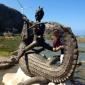# Discussion on: Plane Trigonometry

12 posts / 0 new
Jaywelle T. GalagarDiscussion on: Plane Trigonometry

Following is a discussion on the Reviewer item titled: Plane Trigonometry.

Jaywelle T. Galagara clock has a minute hand 16 cm long and an hour hand 11 cm long. find the distance between the outer tips of the hand at 2:30 o'clock?. . help me

joelitomath1971After sketching a diagram for the problem, you will see that you are dealing with an oblique triangle. Let a=11 cm, b=16 cm, C=105 degrees. You are actually solving for c (the length of the third side of the triangle, which is also the distance between the outer tips of the hands of the clock).
You may now use the Law of Cosines to solve for c. Use the formula: c=a^2+b^2-2ab cos⁡C.
Substituting the values,c =〖(11)〗^2+〖(16)〗^2-2(11)(16) cos⁡〖〖105〗^o 〗. Please figure out what is the final answer. The final unit is in cm. Hope this helps... Good luck!

joelitomath1971I hope this helps...Please post some other problems you might need help with. And I hope you pass your Trig class.

markee22anu bang sagot yan sayo? kasi ang na compute ko ay 26.080 cm

Arnel Corpuz21.635

anmol agarwalAngle b/w the hands = 180-75=105°

Now looking to it as a triangle with 2 sides 16 and 11 cm and angle b/w them as 105°

By cosine formulae

Cos 105=(16^2+ 11^2-x^2)/(2*16*11)

(-0.259)=(377- x^2)/(352)

x^2=468.17

x=21.63

Hence solved

Hope its correct and understood

Arnel Corpuz21.635

Kevin M. EvangelistaLike..

jasmin reyes

hi

Melbourne Barbersir who can help me with my problem in spherical triangle??

Charlon Jay N Unabiasir can you help me with my assignment?

• Mathematics inside the configured delimiters is rendered by MathJax. The default math delimiters are $$...$$ and $...$ for displayed mathematics, and $...$ and $...$ for in-line mathematics.